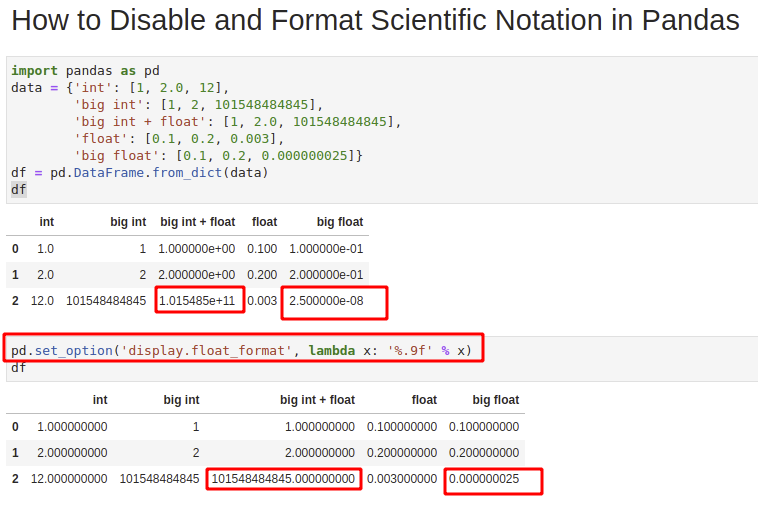Pandas use scientific notation to display float numbers. To disable or format scientific notation in Pandas/Python we will use option `pd.set_option` and other Pandas methods.

All solutions were tested in Jupyter Notebook and JupyterLab.

## Setup

In the post, we'll use the following DataFrame, which is created by the following code:

``````import pandas as pd
data = {'int': [1, 2.0, 12],
'big int': [1, 2, 101548484845],
'big int + float': [1, 2.0, 101548484845],
'float': [0.1, 0.2, 0.003],
'big float': [0.1, 0.2, 0.000000025]}
pd.DataFrame.from_dict(data)
``````

DataFrame looks like:

int big int big int + float float big float
1.0 1 1.000000e+00 0.100 1.000000e-01
2.0 2 2.000000e+00 0.200 2.000000e-01
12.0 101548484845 1.015485e+11 0.003 2.500000e-08

## Step 1: Scientific notation in Pandas

The DataFrame above consists of several columns named depending on the values.

As we can see that some float numbers cause Pandas to display numbers in scientific notation.

Big integer numbers with floats(in the same column) will be displayed in scientific notation too.

The picture below shows both the scientific notation and the normal numbers.## Step 2: Disable scientific notation in Pandas - globally

We can disable scientific notation in Pandas and Python by setting higher float precision:

``````pd.set_option('display.float_format', lambda x: '%.9f' % x)
``````

Now float numbers will be displayed without scientific notation:

int big int big int + float float big float
1.000000000 1 1.000000000 0.100000000 0.100000000
2.000000000 2 2.000000000 0.200000000 0.200000000
12.000000000 101548484845 101548484845.000000000 0.003000000 0.000000025

to reset to the original settings we can use:

``````pd.reset_option('display.float_format', silent=True)
``````

We need to provide the option which will be reset `'display.float_format'`

## Step 3: Format scientific notation in Pandas

If we like to change float number format in Pandas in order to suppress the scientific notation than we can use - `lambda` in combination with formatter '%.9f':

``````df['big float'].apply(lambda x: '%.9f' % x)
``````

Before the change we have:

``````0    1.000000e-01
1    2.000000e-01
2    2.500000e-08
Name: big float, dtype: float64
``````

After the change we will have new float format:

``````0    0.100000000
1    0.200000000
2    0.000000025
Name: big float, dtype: object
``````

As we can see that type is changed from `float64` to `object`. This means that we could say that we (in some way) - convert the scientific notation for float numbers to string.

## Step 4: read_csv and scientific notation in Pandas

Sometimes we may need to use or not scientific notation for `read_csv` in Pandas.

First scientific notation for method `read_csv` depends mostly depends on parameter - `dtype`. This will try to infer float numbers:

``````import pandas
import numpy as np

``````

Once data is read than we can convert or round it by:

``````df = df.apply(pd.to_numeric, errors='coerce')
``````

or round float numbers with method `round`:

``````df.round(5)
``````

This will change the scientific format only for float numbers:

int big int big int + float float big float
1.0 1 1.000000e+00 0.1 0.1
2.0 2 2.000000e+00 0.2 0.2
12.0 101548484845 1.015485e+11 0.003 0.0

## Step 5: Format scientific notation to_html

If we like to disable or format scientific notation in Pandas method `to_html` then we should use parameter - `float_format`:

``````df.to_html(float_format='{:10.9f}'.format)
``````

Now Pandas will generate Data with precision which will show the numbers without the scientific formatting.

Note: `{:10.9f}` can be read as:

• `10` - specifies the total length of the number including the decimal portion
• `9` - is used to specify 9 decimal points

Other examples: `{:30,.18f}` and `{:,.3f}`

## Conclusion

In this post, we covered several examples when we work with scientific notation in Pandas.

Now we can disable or change the format of float numbers shown in scientific notation.